# The third and fourth Zolotarev problems

## Introduction

In 1877, Yegor Ivanovich Zolotarev wrote an article that poses and solves four problems : the first two are about polynomial approximation, while the third and fourth are about rational functions. These problems have become known as Zolotarev's first, second, third, and fourth problems. In this example we focus on Zolotarev's third and fourth problems. Recently, these problems have become important in numerical linear algebra because of a recursive construction of spectral projectors of matrices [3, 4].

## Zolotarev's fourth problem

We start with the Zolotarev's fourth problem as it is perhaps easier than the third problem to visualize. Given two disjoint closed complex sets $E$ and $F$, Zolotarev's fourth problem is to find the rational function $r(x) = p(x)/q(x)$, where $p$ and $q$ are polynomials of degree $k$, that deviates least from the sign function on $E\cup F$, i.e.,

${\rm sgn} (x) = \begin{cases} -1,\quad x\in E,\\ +1,\quad x\in F.\end{cases}$

For general sets $E$ and $F$, the solution to Zolotarev's fourth problem is not known explicitly; however, there are a handful of special cases where the rational function can be given in closed form. The most important special case is when $E$ and $F$ are real disjoint intervals.

For example, if $E = [-b,-1]$ and $F = [1,b]$ with $b>1$ then an explicit solution to Zolotarev's fourth problem is known. It is implemented in RKToolbox's rkfun.gallery command. Here, we plot the extremal rational function $r(x)$ for degree $4$ ($k=4$) and also superimpose on the plot the approximation error:

b = 3;              % E = [-b,-1] and F = [1,b]
k = 4;              % Degree of rational approximant to sign.
r = rkfun.gallery('sign', k/2, b);  % Solution to Z's fourth problem

% Plot the computed rational function:
x = linspace(-5, 5, 1000);
y1 = linspace(-3, -1, 1000); y2 = linspace(1, 3, 1000);
fill([-b -1 -1 -b -b], 1.5*[-1 -1 1 1 -1], .9*[1 1 1] ), hold on
fill([b 1 1 b b], 1.5*[-1 -1 1 1 -1], .9*[1 1 1] )
[~,l1,l2] = plotyy(x, r(x), [y1 0 y2], [(1-abs(r(y1))) NaN (1-abs(r(y2)))]);
l1.LineWidth = 2; l2.LineWidth = 2;
text(-2.1,-1.4,'E','fontsize',16)
text(2,-1.4,'F','fontsize',16)
title('best R_{44} approximant to sign')
xlabel('x'), hold offThe explicit solution of Zolotarev's fourth problem involves Jacobi elliptic functions and complete elliptic integrals. We will not give its formula here, but it can be found scattered throughout the literature (see [1, Sec. 51, Tab. 2, No. 7 & 8]). The MATLAB code in rkfun.gallery uses this explicit formula.

You will notice that the error $|{\rm sgn}(x) - r(x)|$ equioscillates precisely $k+1$ times on both $[-b,-1]$ and $[1,b]$. This verifies the optimality of $r(z)$ [1, Sec. 51]. Since the error equioscillates between a value of $0$ and $1$, there is a number $0<\lambda<1$ such that

$\sup_{x\in [-b,-1]\cup [1,b]} | x - {\rm sgn}(x) | = \frac{1-\lambda}{1+\lambda}.$

The value for $\lambda$ is known to satisfy the equation $k\mu(\lambda) = \mu(1/b)$ [1, Sec. 51], where $\mu$ is the so-called Groetzsch ring function. The Groetzsch ring function $\mu:[0,1]\rightarrow[0,\infty)$ is defined as the ratio of the complete elliptic integral and its complement. It looks like this:

mu = @(lam) pi/2*ellipk( sqrt(1-lam.^2) )./ellipk( lam );
lam = linspace(0, 1, 10000);
vals = zeros(numel(lam), 1);
for j = 1:numel(lam), vals(j) = mu(lam(j)); end
plot(lam, vals, 'linewidth',2), xlabel('\lambda')
title('Groetzsch ring function'), hold off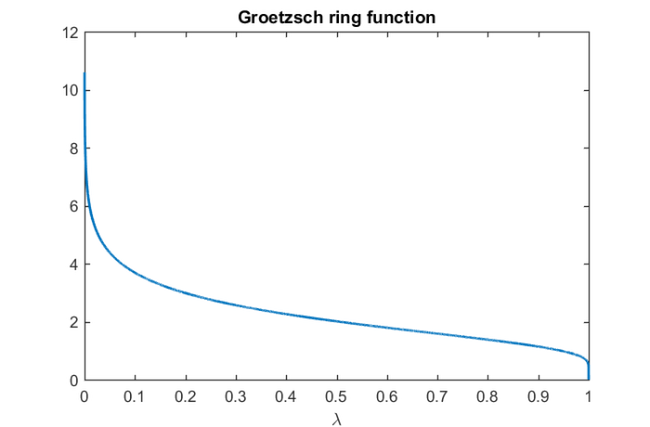Since $\mu$ is a monotonically decreasing function, there is a unique $\lambda$ that solves $k\mu(\lambda) = \mu(1/b)$. We can find it via bisection:

lam1 = 0; lam2 = 1; lam_opt = .5;
target = mu(1/b)/k;
for step = 1:50    % 50 steps finds the root to 16-digits.
lam_opt = mean( [lam1 lam2] );
mid = mu( lam_opt ) - target;
lam1 = lam1 + (mid>0).*(lam_opt - lam1);
lam2 = lam2 + (mid<=0).*(lam_opt - lam2);
end


We can verify that this is the correct $\lambda$ by comparing it to the observed error computed by RKToolbox:

format longe
E4_error_lambda = (1-lam_opt)/(1+lam_opt)
E4_error_observed = max(1-abs(r(y1)))

E4_error_lambda =
1.292026239994933e-03
E4_error_observed =
1.292026239994693e-03


## How well do rational functions approximate the sign function?

Zolotarev's fourth problem shows us that rational functions converge geometrically with respect to the degree $(k,k)$ to the ${\rm sgn}(x)$ function defined on real disjoint intervals. In particular, from these explicit expressions it is known that [2, eqn. (A.5)]

$\sup_{x\in [-b,-1]\cup [1,b]} | r(x) - {\rm sgn}(x) | \leq 4e^{-k\frac{\pi^2}{2\mu(1/b)}},$

which is asymptotically a sharp upper bound. Here, is the computed approximation error $\sup_{x\in [-b,-1]\cup [1,b]}| r(x) - {\rm sgn}(x) |$ and the upper bound above when $b = 100$.

b = 100;                                % E = [-b,-1] and F = [1,b]
y = linspace(-b, -1, 1000);
for k = 2:2:50
r = rkfun.gallery('sign', k/2, b);  % Solution to Z's fourth problem
r_error(k/2) = max(abs(r(y)+1));
end
semilogy(2:2:50, r_error,'.', 'markersize', 30), hold on
semilogy(2:2:40, 4*(exp(pi^2/2/mu(1/b))).^(-(2:2:40)), 'k-', 'linewidth',2)
legend('Computed errors', 'Sharp bound')
xlabel('k'), hold off
hold off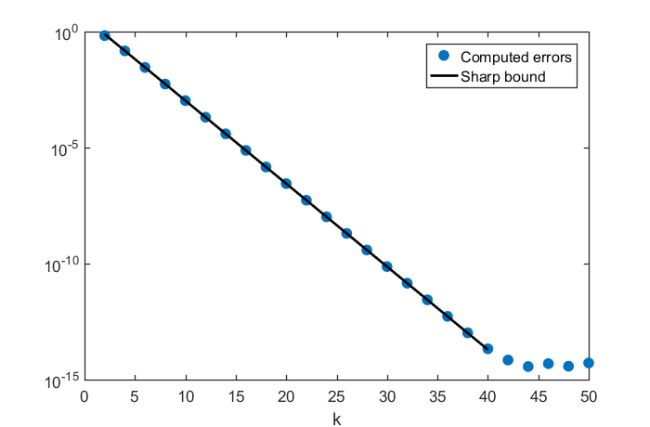The location of the extrema of the error $| r(x) - {\rm sgn}(x) |$ on $[-b,-1]$ and $[1,b]$ are also known explicitly. They are related to the Jacobi elliptic functions. Here, we demonstrate this when $b = 10$.

k = 6;      % rational degree
b = 10;     % sign function on [-10,-1]\cup [1,10]
r = rkfun.gallery('sign', k/2, b);
% Extrema for [-1,-1/b]\cup [1/b,1]:
K = ellipke(1-1/b^2);
[sn, cn, dn] = ellipj((0:k)*K/k, 1-1/b^2);
extrema = b*dn;   % Transplant to [-b,-1]\cup [1,b]

x = linspace(1, b, 1000);
plot(x, r(x), 'linewidth', 2), hold on,
plot(extrema, r(extrema), '.r', 'markersize', 30)
title('extrema of sign approximation error')
xlabel('x'), hold off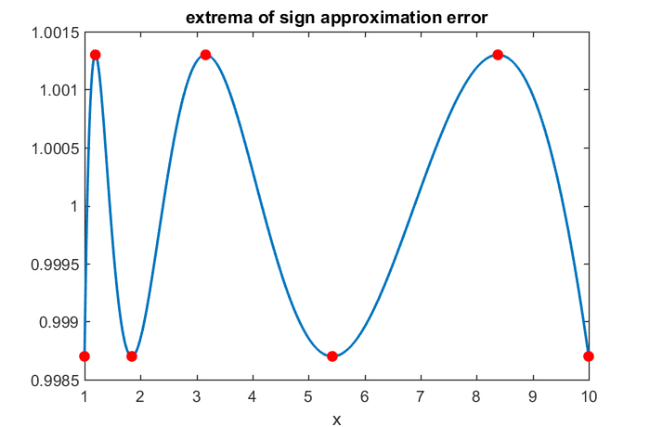## Zolotarev's third problem

Zolotarev's third problem is also related to rational approximation, but this time the problem is to find a rational function that is as small as possible on a set $E$ while being $\geq 1$ in absolute value on another set $F$. More formally, given two disjoint closed complex sets $E$ and $F$, Zolotarev's third problem is to find the rational function $r(x) = p(x)/q(x)$, where $p$ and $q$ polynomials of degree $k$, such that $|r(x)|\geq 1$ for $x\in F$ while $\sup_{x\in E}|r(x)|$ is as small as possible. Therefore, $r(x)$ is the extremal rational function that attains the following infimum:

$Z_k(E,F) = \inf_{r\in R_{kk}} \frac{\sup_{z\in E} |r(z)|}{\inf_{z\in F} |r(z)|},$

where $R_{kk}$ denotes the space of rational functions of degree at most $(k,k)$. Here, the number $Z_k(E,F)$ is referred to as the Zolotarev number.

Again, for general sets $E$ and $F$ the solution to Zolotarev's third problem is not known explicitly; however, when $E = [-b,-1]$ and $F = [1,b]$ are intervals with $b>1$ a closed-form expression is known. In fact, the third and fourth problem are mathematically equivalent (see [1, Sec. 51]). That is, the rational function that solves the fourth problem can be transformed to the solution of the third problem and vice versa. In particular, we have

$\sup_{x\in [-b,-1]\cup [1,b]} | r(x) - {\rm sgn}(x) | = \frac{\sqrt{Z_k(E,F)}}{1+Z_k(E,F)},$

where $E = [-b,-1]$ and $F = [1,b]$.

We can calculate $Z_k([-b,-1],[1,b])$ by using RKToolbox. First, we compute the approximation error between the sign function on $[-b,-1]\cup [1,b]$ and the rational approximation. Then, we solve the equation

$\sup_{[-b,-1]\cup [1,b]}| r(x) - {\mathrm sgn}(x) | = \frac{\sqrt{Z_k(E,F)}}{1+Z_k(E,F)}$

for $Z_k(E,F)$. That is,

vals = 1-r(extrema);
c = mean( vals(1:2:end) );
e = eig( [ 2-4/c^2 1 ; 1 0 ] );
Zk = min(abs(e))

Zk =
4.222581683574948e-07


To further verify the connection between the third and fourth Zolotarev problems we use a Mobius transform to convert the best rational approximation to ${\rm sgn}$ to the extremal rational function for $Z_k(E,F)$.

% Mobius transformation of r(x):
R = @(x) (1 + (1+Zk)/(1-Zk)*r(x))./(1 - (1+Zk)/(1-Zk)*r(x));
x = linspace(-b, b, 5000);
plot(x, R(x), 'linewidth', 2), ylim([-1e4,1e4])
xlabel('x')
title('solution to Zolotarev''s third problem'), hold off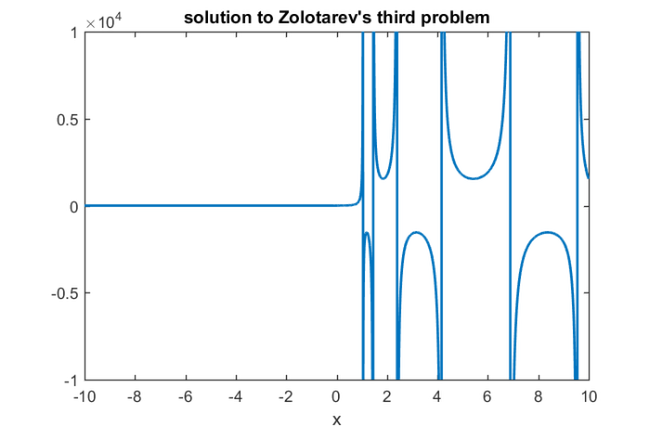One can see that $R(x)$ is such that $|R(x)|\geq 1$ on $F$ while being very small on $E$. We can verify that the rational function $R(x)$ is the extremal rational function by checking that $Z_k(E,F) = \sup_{z\in E} |R(z)|/\inf_{z\in F} |R(z)|$:

x = linspace(-b, -1, 1000);
y = linspace(1, b, 1000);
Zk
max(abs(R(x))) / min(abs(R(y)))

Zk =
4.222581683574948e-07
ans =
4.222581683576213e-07


## Nonsymmetric intervals: Sign approximation

RKToolbox does not directly construct the extremal rational functions to the third and fourth Zolotarev problems on real disjoint intervals that are not symmetric such as $[a,b]\cup [c,d]$ with either $b or $d; however, one can construct it by hand. First, one derives the Mobius transform that transplants $[a,b]\cup [c,d]$ to symmetric intervals of the form $[-\gamma,-1]\cup [1,\gamma]$ with $\gamma>1$. This is only possible when $\gamma$ is selected so the cross-ratios of $(a,b,c,d)$ and $(-\gamma,-1,1,\gamma)$ are equal. (Mobius transforms preserve the cross-ratio of collinear points.) Therefore, we know that $\gamma$ must satisfy

$\left|\frac{(c-a)(d-b)}{(c-b)(d-a)}\right| = \frac{(1+\gamma)^2}{4\gamma}.$

Here, is a graph that checks that the intervals (in blue) are mapped correctly to intervals of the form $[-\gamma,-1]\cup [1,\gamma]$ (in red) by the computed Mobius transform:

a = -10; b = -2; c = 1.1; d = 2*pi;           % [a,b] \cup [c,d]
cross = abs( (c-a)*(d-b)/(c-b)/(d-a) );       % | cross-ratio |
gam = -1 + 2*cross + 2*sqrt(cross^2-cross);   % preserve cross-ratio
% Mobius transform:
B = -(gam+1)*(d-c)/((gam-1)+ 2*(d-c)/(b-c));
A = -2*B/(b-c) - 1; C = 1; D = B;
T = @(z) (A*z + (B - c*A))./(z+(D-c));

% Plot and check:
x = [linspace(a,b) linspace(c,d)];
plot(x+1*1i,'.'), hold on,
plot(T(x)+eps*1i, '.')
xlim([-11 7]), ylim([-.5 1.5])
hold offComposing the Mobius tranform with the best rational approximation to the sign function on $[-\gamma,-1]\cup [1,\gamma]$ derives the best rational approximation on $[a,b]\cup [c,d]$. Here, is the best rational approximation of degree $(4,4)$ on $[-10, -2]\cup[1.1,2\pi]$:

k = 4;
r = rkfun.gallery('sign', k/2, gam);
r = @(z) r( T(z) );
x = linspace(a-2,d+2,1000);
plot(x, r(x), 'linewidth', 2)
xlabel('x'), hold off## Other rational problems in RKToolbox

There are a selection of rational approximation problems that are closely related to Zolotarev's third and fourth problem in RKToolbox. We briefly mention them here as they are provided by the command rkfun.gallery.

Here is the best degree $(8,8)$ rational approximation to the unit step function on $[-1,1]$:

k = 8;
r = rkfun.gallery('step', k/2);
x = linspace(-5, 5, 1000);
plot(x, r(x), 'k-', 'linewidth', 2)
xlabel('x'), hold off
title('best rational approximation to a step function')Here is the best degree $(8,8)$ rational approximation to the sqrt function on $[1,10]$:

k = 8;
r = rkfun.gallery('sqrt', k/2, 10);
x = linspace(1,10,1000);
plot(x, 1 - sqrt(x)./r(x), 'k-', 'linewidth', 2)
xlabel('x'), hold off
title('error in best rational approx to sqrt')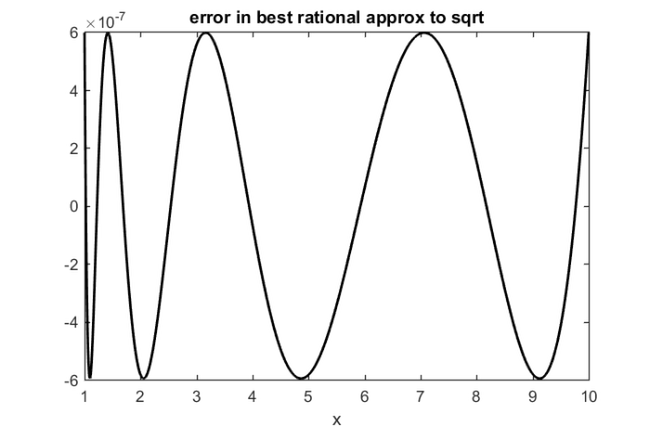Here is the best degree $(8,8)$ rational approximation to the inverse sqrt function on $[1,10]$:

k = 8;
r = rkfun.gallery('invsqrt', k/2, 10);
x = linspace(1,10,1000);
plot(x, 1-r(x).*sqrt(x), 'k-', 'linewidth', 2)
xlabel('x'), hold off
title('error in best rational approx to invsqrt')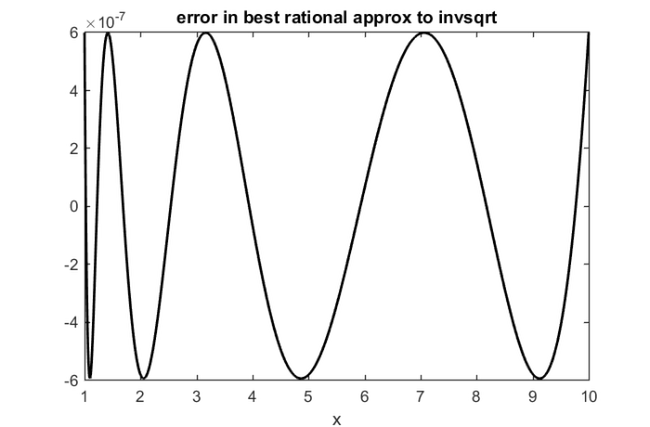## References

 N. I. Akhieser. Elements of the Theory of Elliptic Functions, Transl. of Math. Monographs 79, AMS, Providence RI (1990).

 B. Beckermann and A. Townsend. On the singular values of matrices with displacement structure, SIAM J. Matrix Anal. Appl., 38(4):1227--1248, 2017.

 S. Güttel, E. Polizzi, P. T. P. Tang, and G. Viaud. Zolotarev quadrature rules and load balancing for the FEAST eigensolver, SIAM J. Sci. Comput., 37(4):A2100--A2122, 2015.

 Y. Nakatsukasa and R. W. Freund. Computing fundamental matrix decompositions accurately via the matrix sign function in two iterations: The power of Zolotarev's functions, SIAM Review, 58:461--493, 2016.

 D. I. Zolotarev. Application of elliptic functions to questions of functions deviating least and most from zero, Zap. Imp. Akad. Nauk. St. Petersburg, 30:1--59, 1877.